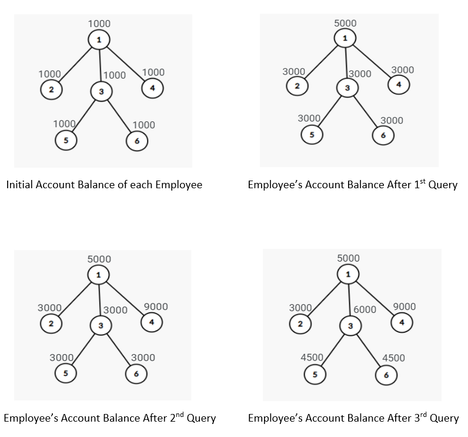# Bonus Projectmaruf089CUET Intra University Jun...
Limits 1s, 256 MB

HOJOBOROLO, a multinational company, has $N$ employees numbered from $1$ through $N$. Each employee, except for lower-level employees, has at least one subordinate. Lower-level employees have no subordinate. Each employee, except for the head of the company, has exactly one direct supervisor. Employee number $1$ is the head of the company and he is in the highest level. There are $N$ teams in the company and employee number $i$ leads the $i$-th team. The $i$-th team consists of the team leader (employee number $i$) and all the direct or indirect subordinates of the team leader.

Now, there will be $Q$ projects this year for the company. The head will distribute all the projects among the teams. One project will not be given to more than one group.

After completing a project, all the team members associated with the project will get a bonus amount which will be added to their account. If employee number $X$ is a team leader then he/she will get a $2Y$ bonus amount and other team members will get a bonus amount of $Y$.

You have to tell the final account balance for each employee at the end of this year.

## Input

The first line contains an integer $T(1 \leq T \leq 10)$, the number of test cases.

The second line contains two integers $N(1 \leq N \leq 5\cdot 10^5 )$ and $Q (1\leq Q \leq N)$, the number of employees and the number of projects.

The third line contains $N$ integers $A_1, A_2, …, A_N (1 \leq A_i \leq 10^3)$, where $A_i$ is the initial account balance of employee number $i$.

Then each of the $N-1$ lines contain two integers $u ( 1 \leq u \leq N )$ and $v (1 \leq v \leq N)$, where there is a connection between $u$ and $v$ and the connection represents the relation between supervisor and subordinate.

The next $Q$ lines describe about the project distribution. In each line there are two integers $X (1 \leq X \leq N)$ and $Y (1 \leq Y \leq 5000)$, the $X$-th team for this project and bonus amount for each subordinates.

It is guaranteed that sum of $N$ over all test cases will not be greater than $5\cdot 10^5$.

## Output

For each test case, print $N$ integers in a line, the final (at the end of the year) account balance of each employee number from $1$ through $N$ respectively.

## Sample

InputOutput
1
6 3
1000 1000 1000 1000 1000 1000
1 2
1 3
1 4
3 6
3 5
1 2000
4 3000
3 1500

5000 3000 6000 9000 4500 4500


After each query, update of the account balance of each employee is given below (Here, each node represents the corresponding employee and his/her current account balance is written nearby) $-$### Submit